# Algebra

Go back to  'Maths'

 1 Introduction to Algebra 2 Algebraic Expressions 3 Algebraic Identities 4 Arithmetic and Geometric Progressions 5 Linear Equations 6 Two-variable Linear Equations 7 Quadratic Equations 8 Exponents, Squares and Cubes 9 Logarithms 10 Sets 11 Polynomials 12 Algebraic Formulas

## Introduction

One evening, constants, variables, and operators were solving math puzzles. They maintained their scores on individual scorecards. A strong wind blew the variables’ scorecards.

The variables were playing really well and did not want to lose. They prayed to Lord Algebra to help them find their scores. Lord Algebra, with the help of some mathematical magic, found the values on the variables’ scorecards.

And, that’s how the story of Algebra began.

In Algebra, the constants are Integers, the operators are the basic arithmetic operators $$(+,-,*,/)$$ and the variables are usually represented by the alphabets $$x$$ and $$y.$$

Example: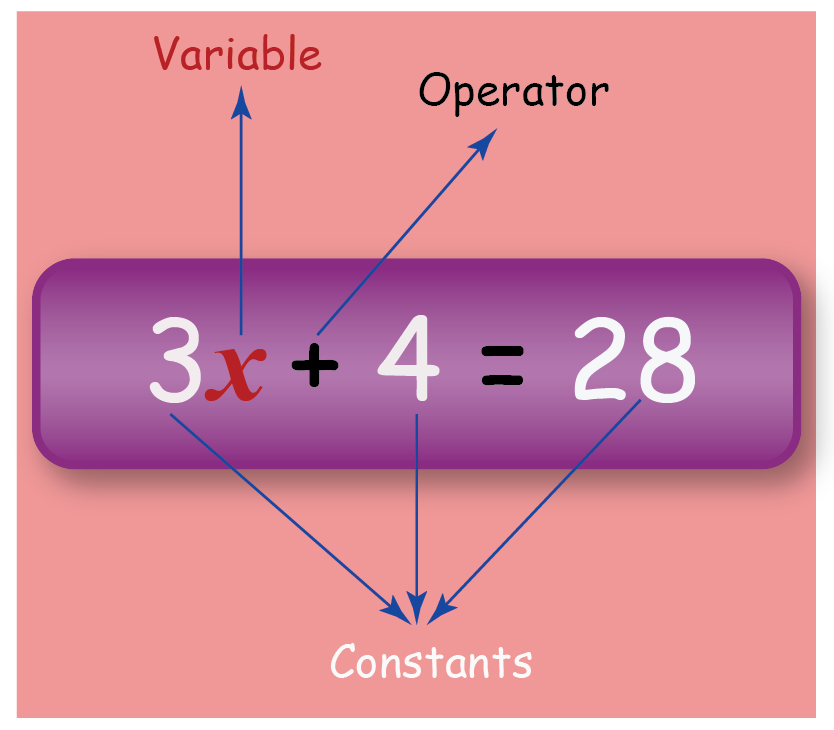You can go ahead and explore all important topics in Algebra by selecting the topics from this list below:

 Algebraic Expressions Quadratic Equations Algebraic Identities Exponents, Squares, Cubes Arithmetic and Geometric Progressions Logarithms Linear Equations Sets Two-Variable Linear Equations Polynomials Algebraic Formulas

## Algebraic Expressions

An algebraic expression is formed using integer constants, variables, and the basic arithmetic operations $$(+, -, x, /).$$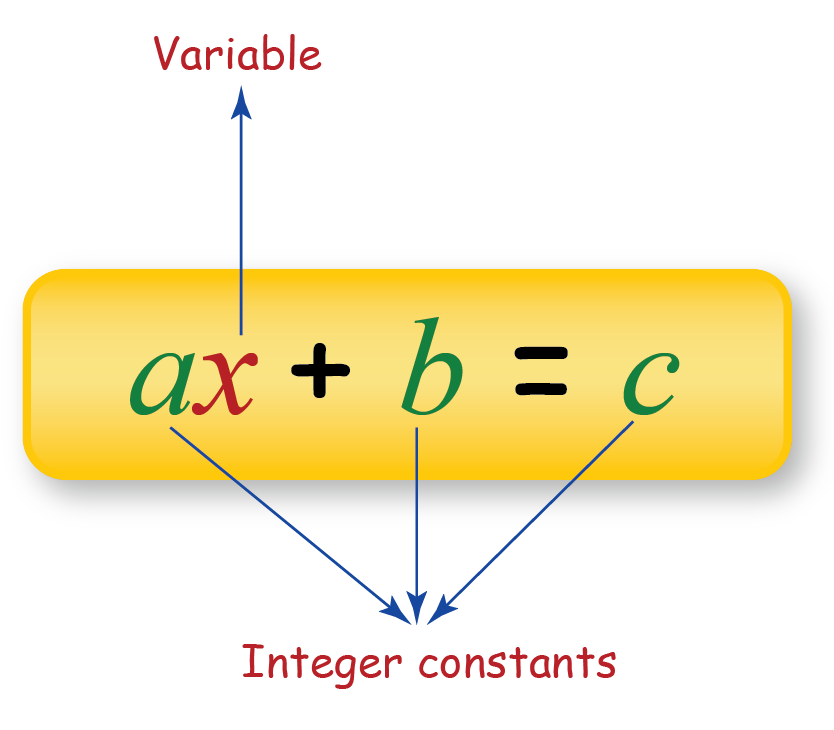Example: $$5x + 6 =16$$

In the above example:

$$x$$ is the variable

$$5,\;6$$ and $$16$$ are the integer constants

$$+$$ is the arithmetic operator being used.

If $$x = 2,$$ then the equation would be $$5 \times 2+6=16.$$

However, if $$x = 3,$$ then the equation would be $$5 \times 3 + 6 = 21.$$

In both the cases, the answer is different as the value of the variable is changed and hence x is called a variable as its value can change.

Algebraic Expressions are introduced in Grade 6.

Algebra is great fun. It comes with equations consisting of constants and variables. These equations can be manipulated with a set of rules. Algebraic equations have a lot of applications in our lives which involve unknown quantities such as measuring distance, financial management, sports, and many more.

In this section, we are going to explore algebra, starting from the basics such as Addition and Subtraction of Algebraic Expressions, Multiplication and Division of Algebraic Expressions, and Factorization of Algebraic expressions.

## Algebraic Identities

An algebraic identity is an equation that is always true regardless of the values assigned to the variables. Identity means that the left-hand side of the equation is identical to the right-hand side, for all values of the variables.

Example: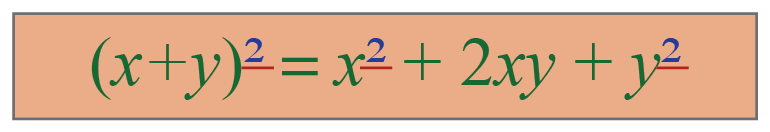Algebraic Identities are introduced in Grade 8.

Let's begin exploring the list of the most important and useful algebraic identities.

## Arithmetic and Geometric Progressions

An arithmetic progression is a sequence of numbers with a definite relationship between successive numbers.

### Arithmetic Progression

An arithmetic progression(AP) is a special type of progression in which the difference between two consecutive terms is always a constant.

Example: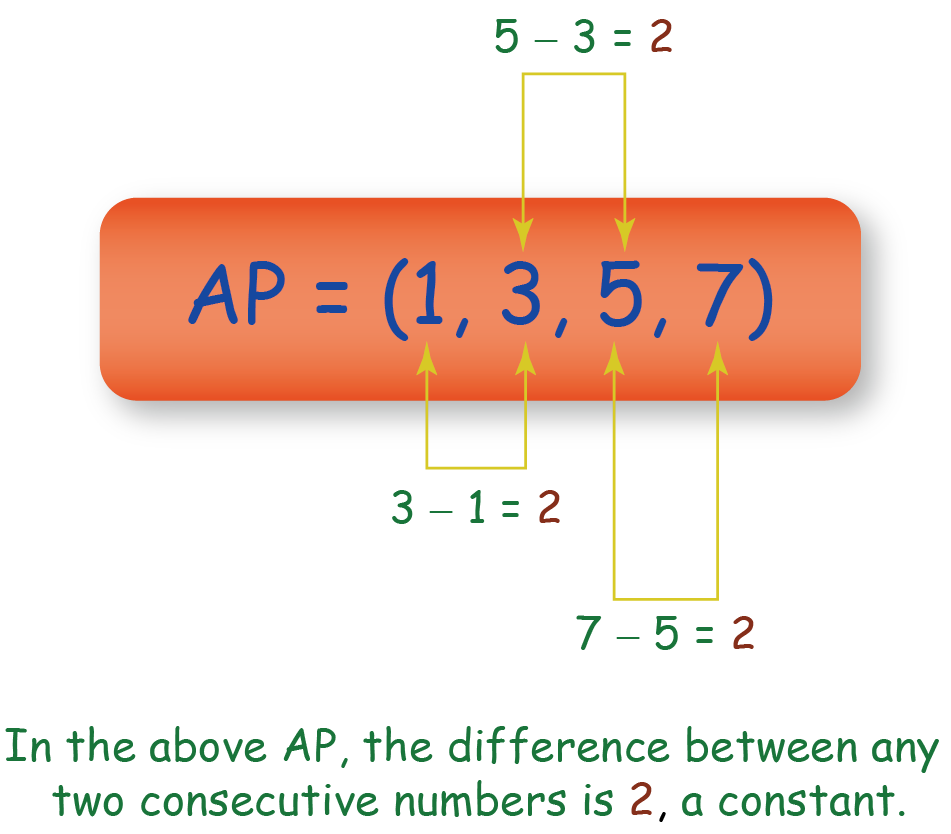### Geometric Progression

Any progression in which the ratio of adjacent terms is fixed is a Geometric Progression or GP.

Example: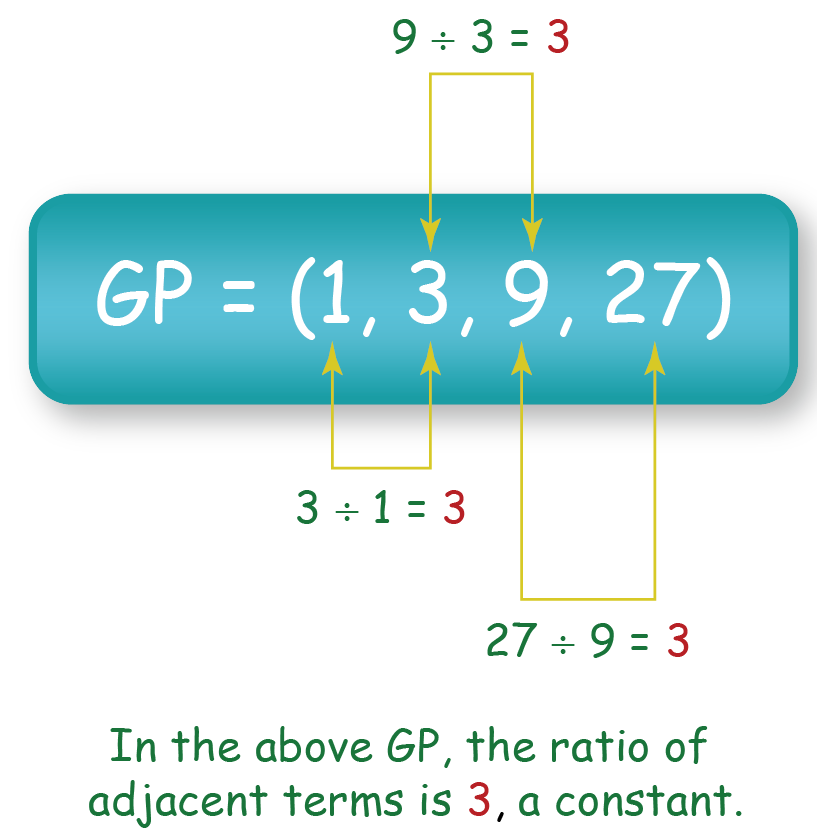Arithmetic and Geometric Progressions are introduced in Grade 10.

You are now familiar with the definition of progression. Once you start learning more about progressions and its types, you will be able to answer questions like What are Progressions, What is an Arithmetic Progression and What are Geometric Progressions. We will also explore concepts like The nth term of an Arithmetic Progression, Sum of n terms of an Arithmetic Progression, The nth term of a Geometric Progression, Sum of n terms of a Geometric Progression and Sum of an Infinite Geometric Progression.

## Linear Equations

A linear equation is an equation between two variables that gives a straight line when plotted on a graph.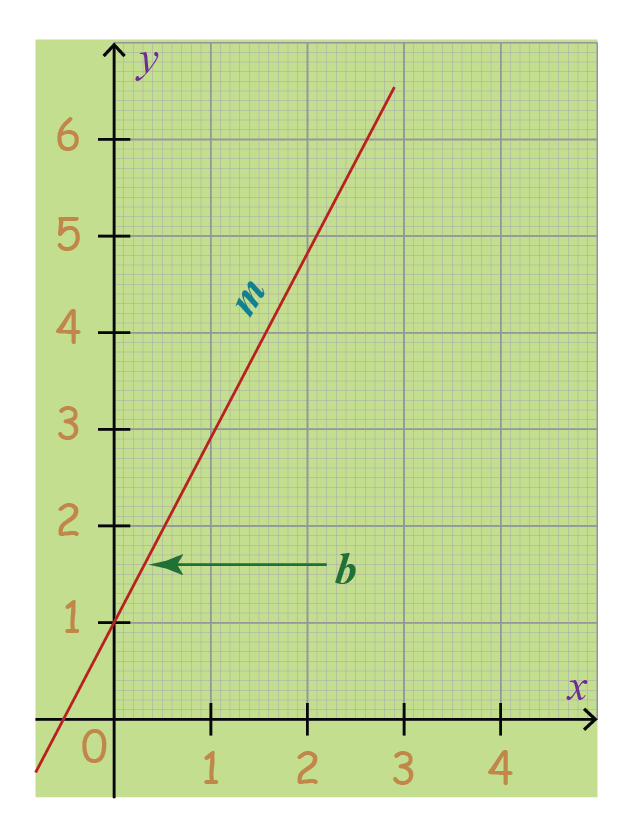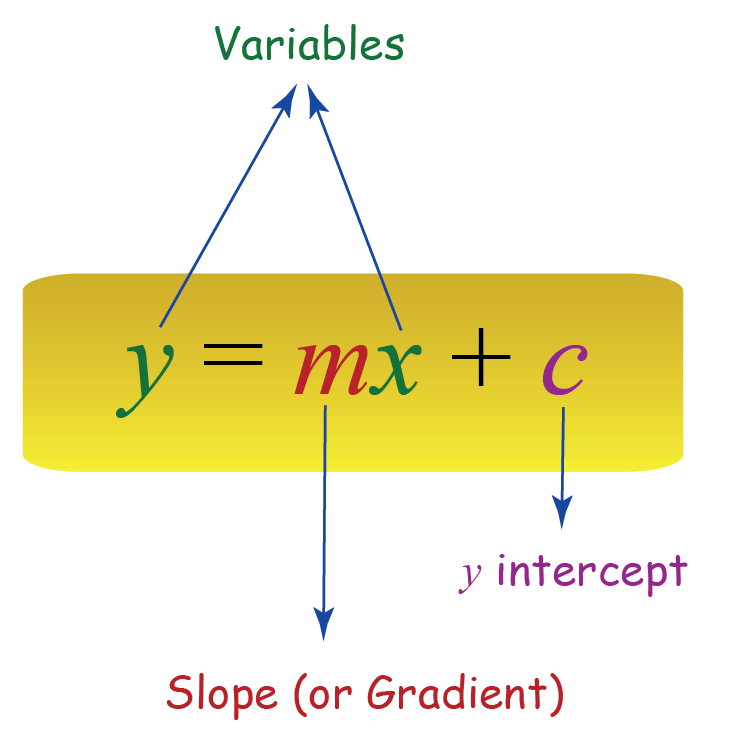Linear Equations are introduced in Grade 7.

Linear Equations are equations consisting of variables whose power is 1. These equations are used in various fields such as demography, archaeology, fractal geometry and many more. In this section, we will learn about the One-Variable Linear Equations and In-equations, and also explore the concepts of Simultaneous Linear Equations.

## Two-variable Linear Equations

An equation that can be put in the form $$ax + by + c = 0,$$ where $$a,$$ $$b$$ and $$c$$ are real numbers and $$a,$$ $$b$$ not equal to zero is called a linear equation in two variables namely $$x$$ and $$y.$$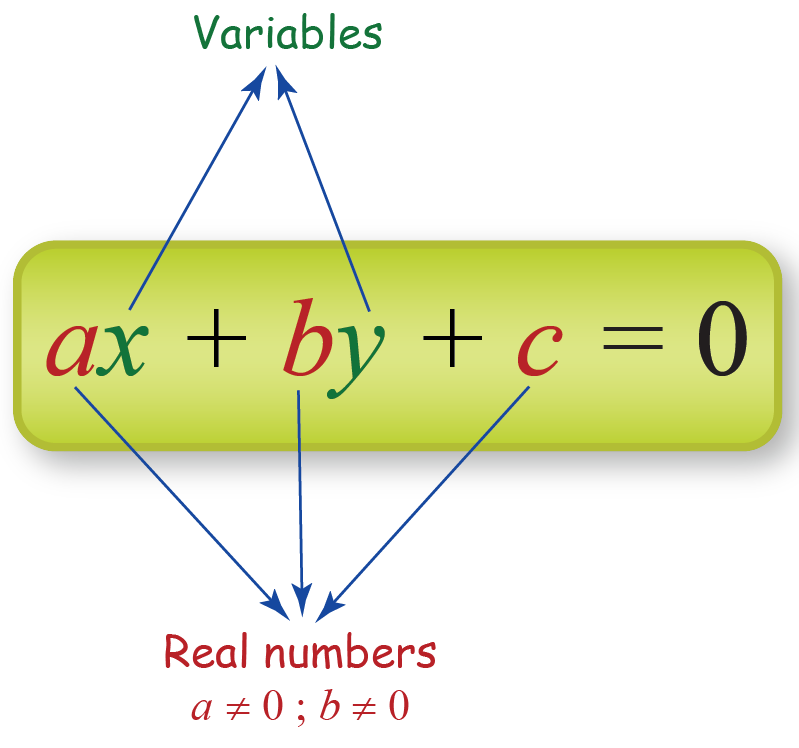Two-Variable Linear Equations are introduced in Grade 9.

We will dive deeper into the linear equations in two variables by exploring more concepts starting from the Introduction to Linear Equations and Inequations and further covering topics such as Solutions of a Linear Equation, Introduction to Graphing, Lines Parallel to Axes, Practical Applications of Linear Equations, Introduction to Linear Inequalities, Linear Inequalities in Two Variables, Linear Equations and Half-Planes, Inequalities involving Absolute Values, Graphically Solving a Pair of Linear Equations, Special Cases in Linear Equations, Overview of Graphical Approach in Linear Equations, Relationships between Coefficients of Nature of Solutions, Substitution Method, Elimination Method, Cross Multiplication Method and Applications of Linear Equations.

A quadratic equation (from the Latin quadratus for "square") can be written in the standard form as: $$ax^2 + bx + c = 0.$$ The values of $$x$$ that satisfy the equation are called solutions of the equation, and a quadratic equation has at most two solutions.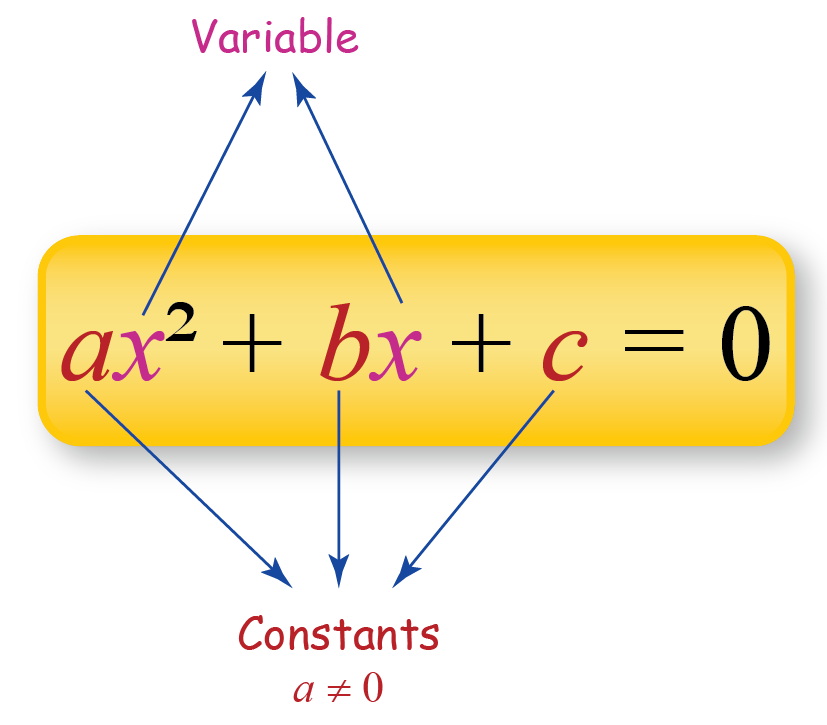We now know how to represent a quadratic equation in the standard form. In this section we will be studying several topics such as Quadratic Expressions, Quadratic Equations, Zeros and Roots, Factorization of Quadratic Equations, Quadratic Formula, Applications of Quadratics, Sum and Product of Roots - Theory, Sum and Product of Roots - Examples, Nature of Roots - Theory, Nature of Roots - Examples, Graphs of Quadratic Expressions - Theory, Graphs of Quadratic Expressions - Examples, Quadratic Inequalities - Theory and Quadratic Inequalities - Examples.

## Exponents, Squares and Cubes

Exponentiation is a mathematical operation, written as $$b^n,$$ involving two numbers, the base $$b$$ and the exponent or power $$n.$$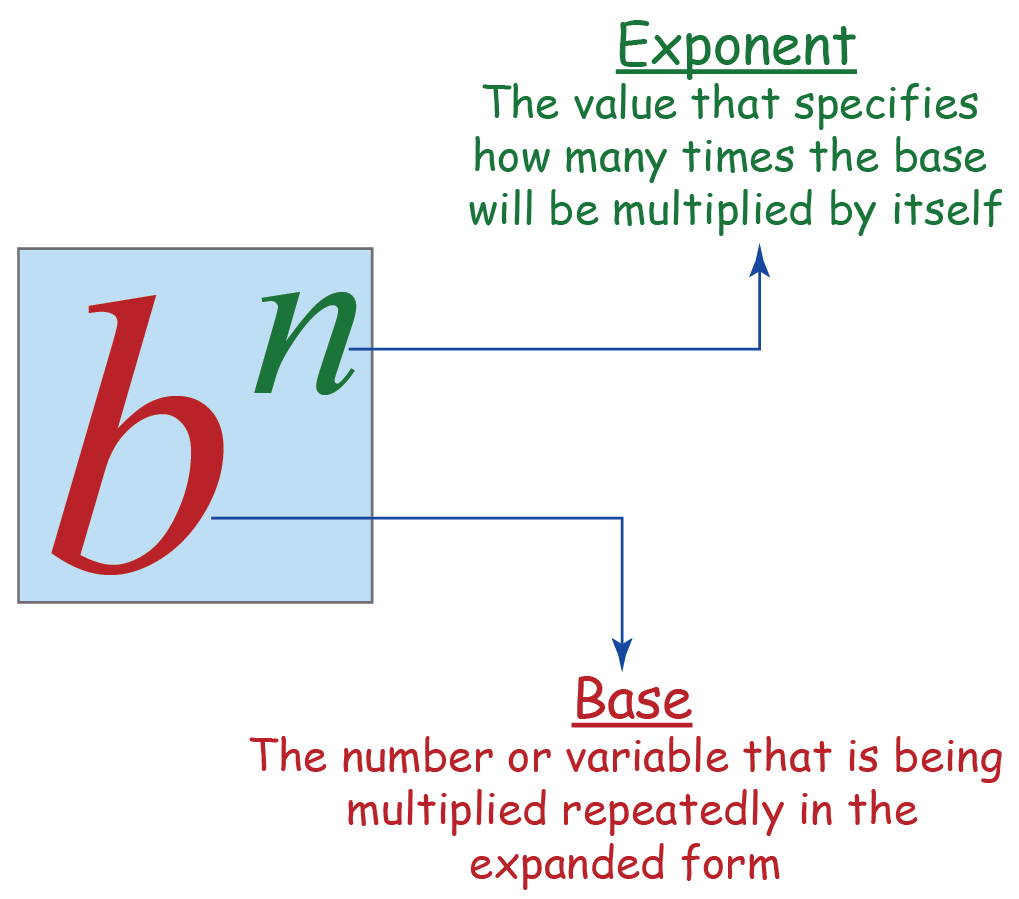Exponents, Squares, Cubes are introduced in Grade 7.

Exponents make it easier to evaluate large numbers. In this section, we are going to learn in detail about Exponents, Squares and Square Roots, Cubes and Cube Roots, Exponential Terms, Multiplying and Dividing Exponential Terms, Non-Integer- Rational Exponents, Irrational Exponents, Constraints on the Base, and Operations on Exponential Terms.

## Logarithms

The logarithm is the inverse function to exponentiation. Logarithms are a convenient way to express large numbers.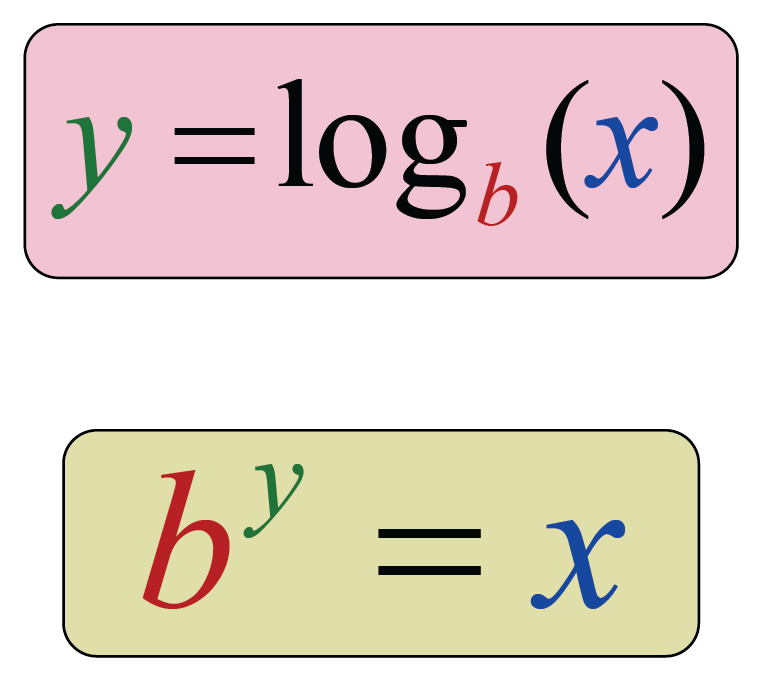Logarithms is introduced in Grade 9.

John Napier discovered the concept of Logarithms in 1614. Logarithms have now become an integral part of modern mathematics and can be used to talk about both tiny and gigantic such as earthquakes, acidity, noise levels etc. In this section, we are going to have a look at the Basic Facts about Logarithms, Properties of Logarithms and Use of Logs in Calculations.

## Sets

A set is a well-defined collection of distinct objects, considered as an object in its own right. The purpose of using sets is to represent the collection of relevant objects in a group.

Example: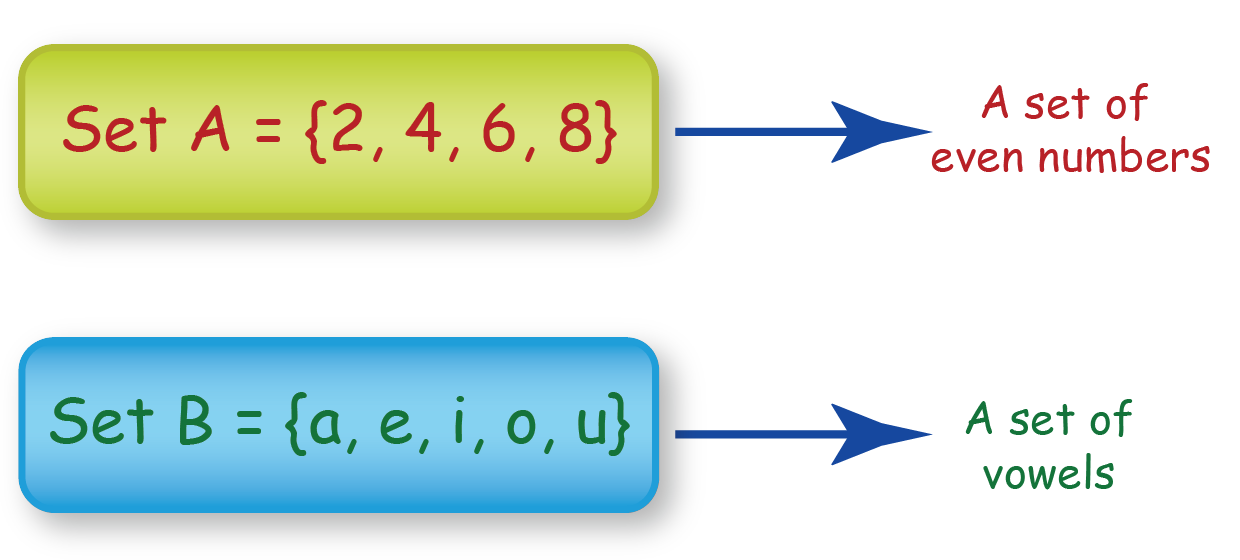Sets are introduced in Grade 6.

Sets are used to represent, collect and study similar data. It has become a fundamental part of modern-day mathematics. Everything nowadays is governed by data and sets help in studying those data. In this section, we will study about Operations on Sets.

## Polynomials

A polynomial is an expression consisting of variables (also called indeterminates) and coefficients, that involves only the operations of addition, subtraction, multiplication, and non-negative integer exponents of variables.

Example: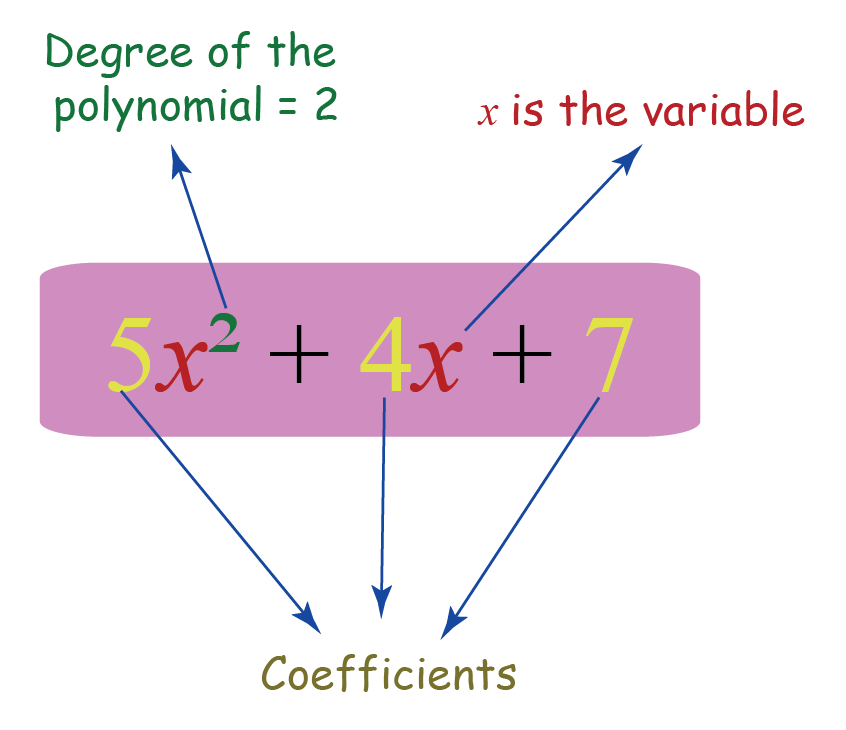Polynomials are introduced in Grade 9.

Polynomial is a special type of mathematical equation and is used in every field of mathematics. Let us understand polynomials better by studying different concepts such as Variables, Constants and Expressions, The Value of an Expression, Equations, Polynomial Expressions, Polynomials in One Variable, Particular Kinds of Polynomials, Degree of a Polynomial, Linear, Quadratic and Cubic Polynomials, Polynomials of Degree n, Addition / Subtraction of Polynomials, Multiplication of Polynomials.

We will also find the answers to questions like What are Zeroes, What are Roots and What is Identity. This section also covers topics like Zero of a Linear Polynomial, Zeros of a Quadratic Polynomial, Zeros of a Cubic Polynomial, Inferring the Locations of Zeroes Indirectly, Calculating Zeros of a Quadratic Polynomial, Division of a Polynomial by a Linear Factor, Division of Polynomials in General, Degree of the Remainder, Division Algorithm - Linear Divisors, The Remainder Theorem, Factors of a Polynomial, Factor Theorem, Factorization of Quadratic Polynomials, Squaring a Trinomial, Cubing a Binomial, An Important Identity, The Importance of Coefficients, Sum and Product of Zeroes in a Quadratic, Zeros of a Cubic, Dividing Two Polynomials, The Degree of the Remainder, The Division Algorithm for Linear Divisors, The Division Algorithm for General Divisors and Tracking Only the Coefficients.

## Algebraic Formulas

The complete list of algebraic formulae involving basic algebraic identities, laws of exponents, and properties of logarithms is listed for easy access.

You can check out the list of all Algebraic Formulas for you to learn and revise.